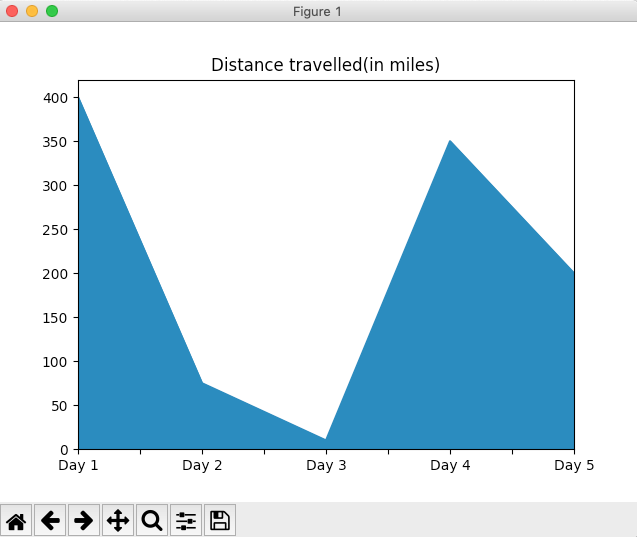# Drawing An Area Plot Using Pandas Series In Python

## Overview:

• An area plot is obtained from a line chart by filling the area between the line and the axis with a colour.
• Compared to the line chart, it is easy to understand the trend of the graph or data using an area plot because of the presence of the coloured area.
• An area plot can be drawn from the data present in a pandas Series using the plot.area() function. Note that plot is a member/attribute of the pandas Series class.
• To draw an area plot for a pandas DataFrame, the area() function can be called on the plot instance of the pandas.DataFrame object.

## Example:

 # Example Python program to draw an Area Plot # for the data present in a pandas.Series object   import pandas as pds import matplotlib.pyplot as plt   # Distance covered on each day as a Python list distanceCovered = [400, 75, 10, 350, 200];   # Create a pandas Series using the Python list series = pds.Series(distanceCovered, index=("Day 1", "Day 2", "Day 3", "Day 4", "Day 5"));   # Draw an area plot series.plot.area(title="Distance travelled(in miles)"); plt.show(block=True);

## Output: